spacing in math mode

# LaTeX spacing in math mode

StartLaTeX faqLaTeX topicsLaTeX course
< text in math mode     > side by side tables

## spacing in math mode

Because blanks were ignored within math mode, they have to be set by a special command:$x\;y$
more commands for spacing in math mode
 $x\!y$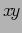$xy$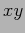$x y$ xy $x\,y$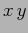$x\:y$$x\;y$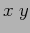$x\quad y$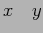$x\qquad y$